# JP2006154979A - Floating point number arithmetic circuit - Google Patents

## Info

Publication number
JP2006154979A
JP2006154979A JP2004341323A JP2004341323A JP2006154979A JP 2006154979 A JP2006154979 A JP 2006154979A JP 2004341323 A JP2004341323 A JP 2004341323A JP 2004341323 A JP2004341323 A JP 2004341323A JP 2006154979 A JP2006154979 A JP 2006154979A
Authority
JP
Japan
Prior art keywords
floating
point number
bit
conversion circuit
instruction
Prior art date
Legal status (The legal status is an assumption and is not a legal conclusion. Google has not performed a legal analysis and makes no representation as to the accuracy of the status listed.)
Pending
Application number
JP2004341323A
Other languages
Japanese (ja)
Inventor
Koichi Hasegawa
Masaaki Ishii
Hiroaki Sakaguchi

Original Assignee
Sony Corp
ソニー株式会社
Priority date (The priority date is an assumption and is not a legal conclusion. Google has not performed a legal analysis and makes no representation as to the accuracy of the date listed.)
Filing date
Publication date
Application filed by Sony Corp, ソニー株式会社 filed Critical Sony Corp
Priority to JP2004341323A priority Critical patent/JP2006154979A/en
Publication of JP2006154979A publication Critical patent/JP2006154979A/en
Pending legal-status Critical Current

• 238000006243 chemical reaction Methods 0 claims description 65
• 230000000694 effects Effects 0 description 5

## Images

•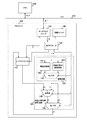•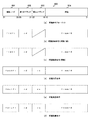•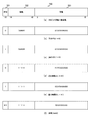•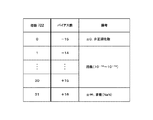••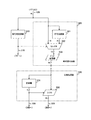•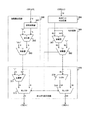•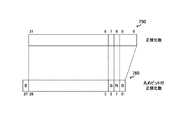•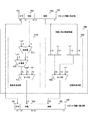•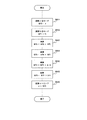•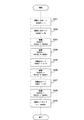## Classifications

• GPHYSICS
• G06COMPUTING; CALCULATING; COUNTING
• G06FELECTRIC DIGITAL DATA PROCESSING
• G06F9/00Arrangements for program control, e.g. control units
• G06F9/06Arrangements for program control, e.g. control units using stored programs, i.e. using an internal store of processing equipment to receive or retain programs
• G06F9/30Arrangements for executing machine instructions, e.g. instruction decode
• G06F9/30003Arrangements for executing specific machine instructions
• G06F9/30007Arrangements for executing specific machine instructions to perform operations on data operands
• G06F9/30029Logical and Boolean instructions, e.g. XOR, NOT
• GPHYSICS
• G06COMPUTING; CALCULATING; COUNTING
• G06FELECTRIC DIGITAL DATA PROCESSING
• G06F7/00Methods or arrangements for processing data by operating upon the order or content of the data handled
• G06F7/38Methods or arrangements for performing computations using exclusively denominational number representation, e.g. using binary, ternary, decimal representation
• G06F7/48Methods or arrangements for performing computations using exclusively denominational number representation, e.g. using binary, ternary, decimal representation using non-contact-making devices, e.g. tube, solid state device; using unspecified devices
• G06F7/483Computations with numbers represented by a non-linear combination of denominational numbers, e.g. rational numbers, logarithmic number system, floating-point numbers
• GPHYSICS
• G06COMPUTING; CALCULATING; COUNTING
• G06FELECTRIC DIGITAL DATA PROCESSING
• G06F9/00Arrangements for program control, e.g. control units
• G06F9/06Arrangements for program control, e.g. control units using stored programs, i.e. using an internal store of processing equipment to receive or retain programs
• G06F9/30Arrangements for executing machine instructions, e.g. instruction decode
• G06F9/30003Arrangements for executing specific machine instructions
• G06F9/30007Arrangements for executing specific machine instructions to perform operations on data operands
• G06F9/3001Arithmetic instructions
• G06F9/30014Arithmetic instructions with variable precision
• GPHYSICS
• G06COMPUTING; CALCULATING; COUNTING
• G06FELECTRIC DIGITAL DATA PROCESSING
• G06F9/00Arrangements for program control, e.g. control units
• G06F9/06Arrangements for program control, e.g. control units using stored programs, i.e. using an internal store of processing equipment to receive or retain programs
• G06F9/30Arrangements for executing machine instructions, e.g. instruction decode
• G06F9/30003Arrangements for executing specific machine instructions
• G06F9/30007Arrangements for executing specific machine instructions to perform operations on data operands
• G06F9/30025Format conversion instructions, e.g. Floating-Point to Integer, decimal conversion
• GPHYSICS
• G06COMPUTING; CALCULATING; COUNTING
• G06FELECTRIC DIGITAL DATA PROCESSING
• G06F9/00Arrangements for program control, e.g. control units
• G06F9/06Arrangements for program control, e.g. control units using stored programs, i.e. using an internal store of processing equipment to receive or retain programs
• G06F9/30Arrangements for executing machine instructions, e.g. instruction decode
• G06F9/30145Instruction analysis, e.g. decoding, instruction word fields
• G06F9/3016Decoding the operand specifier, e.g. specifier format
• G06F9/30167Decoding the operand specifier, e.g. specifier format of immediate specifier, e.g. constants
• GPHYSICS
• G06COMPUTING; CALCULATING; COUNTING
• G06FELECTRIC DIGITAL DATA PROCESSING
• G06F2207/00Indexing scheme relating to methods or arrangements for processing data by operating upon the order or content of the data handled
• G06F2207/38Indexing scheme relating to groups G06F7/38 - G06F7/575
• G06F2207/3804Details
• G06F2207/3808Details concerning the type of numbers or the way they are handled
• G06F2207/3812Devices capable of handling different types of numbers
• G06F2207/3816Accepting numbers of variable word length
• GPHYSICS
• G06COMPUTING; CALCULATING; COUNTING
• G06FELECTRIC DIGITAL DATA PROCESSING
• G06F2207/00Indexing scheme relating to methods or arrangements for processing data by operating upon the order or content of the data handled
• G06F2207/38Indexing scheme relating to groups G06F7/38 - G06F7/575
• G06F2207/3804Details
• G06F2207/3808Details concerning the type of numbers or the way they are handled
• G06F2207/3812Devices capable of handling different types of numbers
• G06F2207/3824Accepting both fixed-point and floating-point numbers

## Abstract

<P>PROBLEM TO BE SOLVED: To efficiently provide arithmetic object data in a floating point number arithmetic circuit. <P>SOLUTION: In this floating point number arithmetic circuit, an instruction with a W bit width is supplied from a memory 200 through a load store unit 110 to an instruction decoder 120. Data with a N bit width embedded in an instruction as an immediate are converted into a floating point number with a F bit width by a converting circuit 130, and defined as an arithmetic object by an arithmetic unit 140 of the floating point number. The data converted by the converting circuit 130 or the arithmetic result calculated by the arithmetic unit are stored through a selector 150 in a register file 170. The converting circuit 130 is provided with an integer converter 300 for converting an integer with the N bit width into a floating point number with the F bit width and a floating point number converter 400 for converting the floating point number with the N bit width into the floating point number with the F bit width, and configured to convert the data with the N bit width into the floating point number with the F bit width. <P>COPYRIGHT: (C)2006,JPO&NCIPI

## Description

The present invention relates to a floating point number arithmetic circuit, and more particularly to a floating point number arithmetic circuit that handles an immediate value and a processor that executes a floating point number instruction with an immediate value.

When data to be calculated is held in a memory, in order to supply the calculation target data to the calculation circuit, it is necessary to read the calculation target data from the memory. In some processors, a field (memory operand) for designating an address of a memory in which operation target data is stored is provided as an operand of an operation instruction so that data on the memory is an operation target.

However, when a memory operand is provided in an operation instruction, it is necessary to access a specified memory address after interpreting the operation instruction, and there is a problem that it takes a long time to complete the data.

Therefore, in a load store architecture represented by recent RISC (Reduced Instruction Set Computer), a data waiting time in one instruction is eliminated by separating a load instruction for reading data from a memory into a register and an operation instruction for executing the operation. In addition, instruction scheduling for speeding up by the compiler can be easily performed. The same architecture is adopted for the instruction set of the arithmetic processor added to the processor (see, for example, Non-Patent Document 1).
"IA-32 Intel (R) Architecture Software Developer's Manual Volume 1: Basic Architecture", Intel Corporation, 2004

Thus, in recent years, an architecture in which a load instruction and an operation instruction are separated has become mainstream. However, in this case, it is necessary to execute a load instruction in addition to an arithmetic instruction, and there are problems that it takes time for memory access and the code size of the program increases.

On the other hand, in the case of integer arithmetic, there is a case where an immediate value field is provided in a part of the field of the arithmetic instruction and the data to be operated is directly embedded in the arithmetic instruction. However, in order to supply floating-point number data to the floating-point number arithmetic circuit, even a single-precision floating-point number requires 32 bits, so it is difficult to embed it as an immediate value in a general 32-bit instruction as an instruction length. is there.

Therefore, an object of the present invention is to efficiently supply operation target data in a floating point number arithmetic circuit.

The present invention has been made to solve the above problems, and a first aspect of the present invention is a floating-point number arithmetic unit that performs a predetermined floating-point number operation on a floating-point number with a predetermined precision, and predetermined data. A floating-point number arithmetic circuit comprising: a conversion circuit that converts the data into a floating-point number with the above precision and supplies the converted floating-point number to at least one input of the floating-point number arithmetic unit. As a result, there is an effect that a calculation of a floating-point number with a predetermined accuracy is executed based on the supplied predetermined data.

According to a second aspect of the present invention, there is provided an instruction decoder for decoding an instruction having an immediate field, and a conversion for converting data contained in the immediate field decoded by the instruction decoder into a floating-point number having a predetermined precision. A circuit, a floating-point arithmetic unit that performs a predetermined floating-point number operation using at least one of the precision floating-point numbers converted by the conversion circuit, and a calculation result by the floating-point number arithmetic unit are stored. And a register. This brings about the effect that the calculation of the floating-point number with a predetermined precision is executed based on the data in the immediate field of the instruction.

In the first or second aspect, the conversion circuit may include an integer converter that converts an integer into the precision floating-point number as the data. As a result, an operation of executing a floating-point number operation with a predetermined precision based on the supplied integer is brought about.

In the first or second aspect, the conversion circuit may include a floating-point number converter that converts a floating-point number with an accuracy different from the accuracy to the floating-point number with the accuracy as the data. . As a result, the floating point number is calculated based on a floating point number different from the accuracy of the arithmetic unit.

In the first or second aspect, the conversion circuit includes an integer converter that converts an integer into the floating-point number with the precision as the data, and a floating-point number with a precision different from the precision as the data. A floating-point number converter for converting to a floating-point number with the above precision and an output of either the integer converter or the floating-point number converter are selected and supplied to at least one input of the floating-point number calculator A conversion selector. As a result, the floating-point number is calculated based on the supplied integer or a floating-point number different from the precision of the arithmetic unit.

The first or second aspect may further include an arithmetic selector that selects and outputs either the output of the floating point number arithmetic unit or the output of the conversion circuit. As a result, there is an effect that a value via the floating point number calculator and a value not via the floating point number calculator are appropriately output as necessary.

According to a third aspect of the present invention, there is provided an instruction decoder for decoding an instruction of W bits (W is an integer of 1 or more) having an immediate field of N bits (N is an integer of 1 or more), and the instruction decoder. A conversion circuit for converting the N-bit data included in the decoded immediate field into an F-bit (F is an integer of 1 or more) floating-point number, and the F-bit floating-point number converted by the conversion circuit A processor comprising: a floating-point number arithmetic unit that performs a predetermined floating-point number operation as at least one input; and a register that stores a calculation result of the floating-point number arithmetic unit. As a result, an operation of executing an F-bit floating point number operation based on N-bit data in the immediate field of the instruction is brought about.

In this third aspect, typically, an N-bit immediate field is a 16-bit immediate field, a W-bit instruction is a 32-bit instruction, and an F-bit floating point number is a 32-bit floating point number. be able to. At this time, the 16-bit immediate field can specify a 16-bit integer or a floating-point number.

According to a fourth aspect of the present invention, there is provided a processor including, as an instruction set, a floating-point arithmetic instruction having an N-bit (N is an integer of 1 or more) immediate field, and the N-bit included in the immediate field. An instruction decoder for extracting data; a conversion circuit for converting the extracted N-bit data into an F-bit (F is an integer of 1 or more) floating-point number; and the F-bit floating point converted by the conversion circuit A processor comprising a floating-point number computing unit that performs a predetermined floating-point number calculation with at least one decimal point as an input. As a result, it is possible to provide an immediate field in the floating-point arithmetic instruction, which brings about the effect of improving the utilization efficiency of the program code, the memory and the like.

In this fourth aspect, as a typical example, an N-bit immediate field can be a 16-bit immediate field, and an F-bit floating point number can be a 32-bit floating point number.

According to the present invention, it is possible to achieve an excellent effect of efficiently supplying operation target data in a floating point number arithmetic circuit.

Next, embodiments of the present invention will be described in detail with reference to the drawings.

FIG. 1 is a diagram showing a configuration example of the processor 100 according to the embodiment of the present invention. The processor 100 is connected to the memory 200 via a bus 210, and includes a load / store unit 110, an instruction decoder 120, a floating point number arithmetic circuit 160, a register file 170, and a control unit 190.

The load / store unit 110 reads a W-bit instruction (W is an integer of 1 or more) or F-bit (F is an integer of 1 or more) floating-point number data from the memory 200, or reads F-bit data from the memory 200. Writes floating point number data.

The instruction decoder 120 receives a W-bit instruction from the load / store unit 110 and performs decoding according to the instruction format. The decoding result in the instruction decoder 120 is transmitted to each part of the processor 100 as a control signal.

The floating-point number arithmetic circuit 160 is supplied with N-bit (N is an integer of 1 or more) data from the instruction decoder 120 and F-bit floating-point number data from the register file 170, and performs a predetermined calculation to perform F Output bit data.

The register file 170 is a register that holds M pieces of F-bit floating point number data (M is an integer of 1 or more). The register file 170 performs writing or reading with the load / store unit 110 or the floating-point number arithmetic circuit 160. The type of access to which data in the register file 170 is controlled based on the decoding result by the instruction decoder 120.

The control unit 190 is used to control each hardware module in the processor 100.

Here, the floating point number arithmetic circuit 160 further includes a conversion circuit 130, an arithmetic unit 140, and a selector 150. The conversion circuit 130 converts the N-bit data from the instruction decoder 120 into F-bit floating point number data as an integer, and the N-bit data from the instruction decoder 120 as an N-bit floating point number as an F-bit floating point number. A floating-point number converter 400 that converts to numerical data and a selector 135 that selects the output of either the integer converter 300 or the floating-point number converter 400 are provided. The arithmetic unit 140 is an arithmetic unit that calculates F-bit floating point number data. The selector 150 selects either the output of the arithmetic unit 140 or the output of the conversion circuit 130 and outputs it to the register file 170.

In FIG. 1, the instruction length is W bits, the floating point number data width is F bits, and the data width supplied from the instruction decoder 120 is N bits. Assume that the decimal point data width is 32 bits each. Further, it is assumed that the data width supplied from the instruction decoder 120 is 16 bits. However, the present invention is not limited to this, and N-bit data in a W-bit instruction length can be appropriately converted into F-bit floating point number data.

FIG. 2 is a diagram showing an instruction format of an immediate instruction according to the embodiment of the present invention. Referring to FIG. 2A, this immediate instruction is a 32-bit instruction, and each field of a 6-bit function code 801, a 5-bit first operand 802, a 5-bit second operand 803, and a 16-bit immediate value 804 It has.

The function code 801 is a field representing the operation code of the instruction. The first and second operands 802 and 803 are operands of the instruction, and the second operand 803 may not exist depending on the instruction. The immediate value 804 supplies a 16-bit integer or a 16-bit floating point number as an immediate value.

Referring to FIGS. 2B to 2F, as an example of an instruction in the immediate instruction format, an immediate storage instruction (floating point), an immediate storage instruction (integer), an immediate addition instruction, an immediate multiplication instruction, and an immediate division instruction It is shown.

In the immediate storage instruction (floating point number) of FIG. 2B, “fldfi” is specified as the function code 801 and the register “rd” is specified as the first operand 802 as the operand. In the immediate value 804, a 16-bit floating point number (fimm16) is designated. By executing this immediate storage instruction (floating point number), the 16-bit floating point number is converted into a 32-bit floating point number by the floating point number converter 400 and stored in the register rd via the selectors 135 and 150.

In the immediate storage instruction (integer) in FIG. 2C, “fldi” is specified as the function code 801 and the register “rd” is specified as the first operand 802 as the operand. A 16-bit integer (imm16) is designated as the immediate value 804. By executing this immediate storage instruction (integer), the 16-bit integer is converted into a 32-bit floating point number by the integer converter 300 and stored in the register rd via the selectors 135 and 150.

In the immediate addition instruction of FIG. 2D, “faddfi” is specified as the function code 801, the register “rd” is specified as the first operand 802, and the register “rs” is specified as the second operand 803. . In the immediate value 804, a 16-bit floating point number (fimm16) is designated. By executing this immediate addition instruction, the 16-bit floating point number is converted into a 32-bit floating point number by the floating point number converter 400 and supplied to one input of the arithmetic unit 140 via the selector 135. Then, the arithmetic unit 140 performs addition with the register rs, and the addition result is stored in the register rd via the selector 150.

In the immediate multiplication instruction of FIG. 2E, “fmulfi” is specified as the function code 801, the register “rd” is specified as the first operand 802, and the register “rs” is specified as the second operand 803. . In the immediate value 804, a 16-bit floating point number (fimm16) is designated. By executing this immediate multiplication instruction, the 16-bit floating point number is converted into a 32-bit floating point number by the floating point number converter 400 and supplied to one input of the arithmetic unit 140 via the selector 135. Then, the arithmetic unit 140 performs multiplication with the register rs, and the multiplication result is stored in the register rd via the selector 150.

In the immediate division instruction of FIG. 2F, “fdivfi” is specified as the function code 801, the register “rd” is specified as the first operand 802, and the register “rs” is specified as the second operand 803. . In the immediate value 804, a 16-bit floating point number (fimm16) is designated. By executing this immediate division instruction, the 16-bit floating point number is converted into a 32-bit floating point number by the floating point number converter 400 and supplied to one input of the arithmetic unit 140 via the selector 135. Then, in the arithmetic unit 140, the register rs is divided by the conversion result, and the division result is stored in the register rd via the selector 150.

FIG. 3 is a diagram showing a format example of a 16-bit floating point number in the embodiment of the present invention. Referring to FIG. 3A, in this format example, a 1-bit sign part 721, a 5-bit exponent part 722, and a 10-bit mantissa part 723 are shown.

The sign part 721 represents a sign of a numerical value, and stores either “1” representing positive or “0” representing negative.

The exponent part 722 represents an exponent having “10” as a radix, and has a bias expression in which “15” is added. That is, as shown in FIG. 4, in the value before being biased, an integer from “−14” to “+15” representing an exponent, positive infinity (+ ∞), negative infinity (−∞) Or “+16” representing a non-number (NaN), positive zero (+0), negative zero (−0), or “−15” representing a denormalized number is stored. The

The mantissa part 723 represents a mantissa normalized with “2” as a radix, and stores a value in which the most significant bit is omitted after normalization. However, in a 16-bit floating point number, a positive denormalized number is treated as positive zero (+0), and a negative denormalized number is treated as negative zero (−0). As shown in FIG. 3B or FIG. 3C, when the sign part 721 is “0” or “1” and the exponent part 722 and the mantissa part 723 are all “0” in a 16-bit floating point number, positive zero Represents (+0) or negative zero (−0).

Further, as shown in FIG. 3D or FIG. 3E, in the 16-bit floating point number, the sign part 721 is set to “0” or “1”, the exponent part 722 is set to “11111”, and the mantissa part 723 is all set to “0”. "Represents positive infinity (+ ∞) or negative infinity (−∞). Further, as shown in FIG. 3F, in the 16-bit floating point number, when the sign part 721 is “0” or “1”, the exponent part 722 is “11111”, and the mantissa part 723 is a value other than “0”. Represents a non-number (NaN).

Here, the explanation has been made assuming that the exponent part 722 has 5 bits and the mantissa part 723 has 10 bits. However, the present invention is not limited to this, and other combinations of bit numbers may be used.

FIG. 5 is a diagram illustrating a configuration example of the integer converter 300 according to the embodiment of the present invention. The integer converter 300 converts a 16-bit integer 710 embedded as an immediate value 804 in an immediate instruction 800 into an IEEE754 single-precision (32-bit) floating point number 790. The integer converter 300 includes a sign determination circuit 310, an absolute value conversion circuit 320, a normalization circuit 330, an exponent calculation circuit 340, a rounding bit addition circuit 350, a rounding circuit 360, and a carry correction circuit 370. The bit truncation circuit 380 is provided. It is assumed that the rounding mode in the integer converter 300 conforms to IEEE 754 RN (Round to Nearest).

The sign determination circuit 310 determines the sign of the signed integer 711 in the 16-bit integer 710 supplied by the signal line 129, and the determination result is the sign 791 in the 32-bit floating point number 790 via the signal line 319. Supplied as The determination result is also used for sign inversion in the absolute value conversion circuit 320.

The absolute value conversion circuit 320 outputs the absolute value of the signed integer 711 in the 16-bit integer 710 supplied by the signal line 129 as a 32-bit absolute value. Referring to FIG. 6, the absolute value conversion circuit 320 includes a sign inverter 321, a selector 322, and a bit expander 323. The sign inverter 321 inverts the sign of the signed integer 711 in the 16-bit integer 710 supplied by the signal line 129. The selector 322 selects the input or output of the sign inverter 321 based on the determination result of the sign determination circuit 310 and outputs the absolute value of the signed integer 711. The bit expander 323 extends the 16-bit absolute value output from the selector 322 to a 32-bit absolute value.

The normalization circuit 330 outputs a normalized number obtained by normalizing the 32-bit absolute value output from the absolute value conversion circuit 320. Referring to FIG. 6, the normalization circuit 330 includes a counter 331 and a shifter 332. The counter 331 counts the number of “0” s that are continuous from the most significant bit to the least significant bit of the 32-bit absolute value output from the absolute value conversion circuit 320. The shifter 332 shifts the 32-bit absolute value output from the absolute value conversion circuit 320 to the left by using the count result of the counter 331 as a shift amount, and outputs it as a 32-bit normalized number. The normalized number obtained in this way is supplied to the rounding bit adding circuit 350 through the signal line 339, and the counting result by the counter 331 is supplied to the exponent calculation circuit 340 through the signal line 338.

The exponent calculation circuit 340 calculates the exponent of the normalized number generated by the normalization circuit 330. Referring to FIG. 7, the exponent calculation circuit 340 includes a sign inverter 341, an adder 342, and an adder 343. The sign inverter 341 inverts the sign of the count result of the shift number supplied from the counter 331 via the signal line 338. As a result, the output of the adder 342 is obtained by subtracting the shift count counting result from “31”. The adder 343 adds “127” to the subtraction result. Thereby, the exponent of the normalized number is calculated. The exponent thus calculated is supplied to the adder 371 and the selector 372 in the carry correction circuit 370.

The rounding bit adding circuit 350 generates a normalized number with rounding bits of the normalized number generated by the normalizing circuit 330. Referring to FIG. 8, the normalized number 750 generated by the normalizing circuit 330 is set as a normalized number 760 with a rounding bit as follows. That is, bits 31 to 8 of the normalized number 750 become bits 26 to 3 of the normalized number 760 with rounding bits. Bit 7 of normalized number 750 becomes Guard bit 2 of normalized number 760 with a rounding bit. Bit 6 of normalized number 750 becomes Round bit 1 of normalized number 760 with a rounding bit. The logical sum of bits 5 to 0 of the normalized number 750 is the Sticky bit 0 of the normalized number 760 with a rounding bit. Further, “0” is set in the most significant bit 27 of the normalized number 760 with a rounding bit so that the rounding circuit 360 can detect a carry.

Referring to FIG. 7 again, the rounding circuit 360 performs rounding processing, and includes adders 361 and 362. The adder 361 adds the value of bit 3 to the normalized number 760 with a rounding bit by the rounding bit addition circuit 350. The adder 362 further adds “3” to the addition result from the adder 361. The addition result is supplied as data to the shifter 373 and the selector 374 in the carry correction circuit 370, and the presence / absence of a carry in the addition is used as carry information as a selection signal for the selectors 372 and 374 in the carry correction circuit 370. Supplied.

The carry correction circuit 370 corrects the exponent calculated by the exponent calculation circuit 340 and the normalized number rounded by the rounding circuit 360. The carry correction circuit 370 includes an adder 371, a selector 372, a shifter 373, and a selector 374. The adder 371 adds “1” to the exponent calculated by the exponent calculation circuit 340. The selector 372 selects the output of the adder 371 if a carry has occurred in the adder 362, and selects the exponent from the exponent calculation circuit 340 if no carry has occurred. The output of the selector 372 is supplied as an index 792 in a 32-bit floating point number 790 via a signal line 378.

The shifter 373 shifts the normalized number from the rounding circuit 360 to the right by 1 bit. The selector 374 selects the output of the shifter 373 if a carry has occurred in the adder 362 and selects the normalized number from the rounding circuit 360 if no carry has occurred. The output of the selector 374 is supplied to the bit truncation circuit 380 via the signal line 379.

Referring to FIG. 5 again, the bit truncation circuit 380 generates a 23-bit mantissa by truncating bits 27, 26, and 2 to 0 from the rounded normalized number supplied from the carry correction circuit 370. . The output of the bit truncation circuit 380 is supplied as a mantissa 793 in a 32-bit floating point number 790.

FIG. 9 is a diagram illustrating a configuration example of the floating-point number converter 400 according to the embodiment of the present invention. This floating point number converter 400 converts a 16-bit floating point number 720 into a 32-bit floating point number 790, and includes an exponent conversion circuit 410 and a mantissa conversion circuit 420.

The exponent conversion circuit 410 converts the exponent 722 in the 16-bit floating point number 720 into the exponent 792 of the 32-bit floating point number 790, and includes adders 411 and 412 and a selector 413. The adder 411 subtracts “15” which is a bias value of the 16-bit floating point number 720. The adder 412 adds 127 which is a bias value of the 32-bit floating point number 790 to the addition result by the adder 411.

The selector 413 selects one of the addition result by the adder 412 and the number “255” or the number “0” according to the contents of the 16-bit floating point number 720. That is, if the 16-bit floating point number 720 is any of positive infinity (+ ∞), negative infinity (−∞), or non-number (NaN), the exponent 792 of the 32-bit floating point number 790 is obtained. “255” representing infinity or non-number is selected. If the 16-bit floating point number 720 is positive zero (+0), negative zero (−0), or a denormalized number, “0” representing zero as the exponent 792 of the 32-bit floating point number 790. Select. If it is a floating point number other than these, the addition result by the adder 412 is selected as the exponent 792 of the 32-bit floating point number 790.

The mantissa conversion circuit 420 converts the mantissa 723 in the 16-bit floating point number 720 into the mantissa 793 of the 32-bit floating point number 790, and includes a selector 423. According to the contents of the 16-bit floating point number 720, the selector 423 is a number obtained by supplementing 13 bits “0” in the lower order of the 10-bit mantissa 723 and 13 bits “0” in the higher order of the 10-bit mantissa 723. Either a number supplemented by or a 23-bit “0” is selected. That is, if the 16-bit floating point number 720 is any of positive infinity (+ ∞), negative infinity (−∞), or non-number (NaN), the mantissa 793 of the 32-bit floating point number 790 is A number obtained by supplementing 13 bits of “0” above the 10-bit mantissa 723 is selected. If the 16-bit floating point number 720 is positive zero (+0), negative zero (−0), or a denormalized number, the mantissa 793 of the 32-bit floating point number 790 is 23 bits “0”. Select. For other floating-point numbers, a number in which a 13-bit “0” is added to the lower order of the 10-bit mantissa 723 is selected as the mantissa 793 of the 32-bit floating-point number 790.

In this floating point number converter 400, the code 721 of the 16-bit floating point number 720 is used as it is as the code 791 of the 32-bit floating point number 790.

FIG. 10 is a diagram showing an example of a program code string in the embodiment of the present invention. Here, a program for obtaining the volume of a cone is given as an example. This is to obtain the volume v of the cone based on the radius r and the height h by the following equation.
v ← (π × r 2 × h) / 3

First, the contents of the memory area of the variable r are loaded into the register \$ f0 (step S911). Further, the contents of the memory area of the variable h are loaded into the register \$ f1 (step S912). Then, the multiplication of the register \$ f0 and the register \$ f0 is executed, and the multiplication result is stored in the register \$ f0 (step S913). This is to calculate the square of the radius r.

Subsequently, the multiplication of the register \$ f0 and the register \$ f1 is executed, and the multiplication result is stored in the register \$ f0 (step S914). This is to multiply the square of the radius r by the height h.

Then, the multiplication of the register \$ f0 and the immediate value 3.14 is executed, and the multiplication result is stored in the register \$ f0 (step S916). Here, the immediate multiplication instruction (fmulfi) described in FIG. 2E can be used. That is, by setting both the first and second operands 802 and 803 to the register \$ f0 and the immediate value 804 to 16 bits of “3.14”, a desired operation can be realized with one instruction.

Subsequently, division is performed by dividing the register \$ f0 by the immediate value 3.0, and the division result is stored in the register \$ f0 (step S918). Here, the immediate division instruction (fdivfi) described in FIG. 2F can be used. That is, by setting both the first and second operands 802 and 803 as the register \$ f0 and the immediate value 804 as 16 bits “3.0”, a desired operation can be realized with one instruction.

Finally, the contents of the register \$ f0 are stored in the memory area of the variable v (step S919). Thereby, the calculation result of the volume of the cone is stored in the memory area of the variable v.

FIG. 11 is a diagram showing an example of a program code string in the prior art. Here, the SSE instruction group (Streaming SIMD Extensions instructions) in Non-Patent Document 1 is used as an example of the instruction set. The content of the program is to obtain the volume of the cone as in the case of FIG.

First, the contents of the memory area of the variable r are loaded into the register XMM0 (step S921). Further, the contents of the memory area of the variable h are loaded into the register XMM1 (step S922). Then, multiplication between the register XMM0 and the register XMM0 is executed, and the multiplication result is stored in the register XMM0 (step S923). This is to calculate the square of the radius r.

Subsequently, multiplication of the register XMM0 and the register XMM1 is executed, and the multiplication result is stored in the register XMM0 (step S924). This is to multiply the square of the radius r by the height h.

Then, the contents of the memory area holding the constant pi (3.14) are loaded into the register XMM1 (step S925). Thereafter, multiplication of the register XMM0 and the register XMM1 is executed, and the multiplication result is stored in the register XMM0 (step S926).

Subsequently, the contents of the memory area in which the constant dv (3.0) is held are loaded into the register XMM1 (step S927). Thereafter, division is performed by dividing the register XMM0 by the register XMM1, and the division result is stored in the register XMM0 (step S928).

Finally, the contents of the register XMM0 are stored in the memory area of the variable v (step S929). Thereby, the calculation result of the volume of the cone is stored in the memory area of the variable v.

Here, comparing FIG. 10 with FIG. 11, it can be seen that the prior art of FIG. 11 requires an extra instruction for loading constants from the memory in steps S925 and S927. In the embodiment of the present invention shown in FIG. 10, since a constant is embedded as an immediate value in a multiplication instruction (step S916) and a division instruction (step S918), a load instruction becomes unnecessary and the program code is shortened.

As described above, according to the embodiment of the present invention, the floating-point arithmetic circuit 160 converts the 16-bit integer 710 into the 32-bit floating-point number 790, and the 32-bit floating-point number 720 into 32. By providing the floating point number converter 400 that converts the bit floating point number 790, the 16-bit immediate value 804 in the immediate instruction 800 can be converted into a 32-bit floating point number 790 and desired processing can be performed.

More specifically, first, the code size of the program can be reduced by reducing load instructions for loading numerical data from the memory. Second, since the load instruction is deleted, there is no need to wait for data from the memory, and the utilization efficiency of the floating point number arithmetic circuit can be improved. Thirdly, by deleting the load instruction, the number of memory accesses can be reduced, and the utilization efficiency of the bus between the arithmetic circuit and the memory can be improved. Fourthly, the memory utilization efficiency can be improved by embedding a floating point number originally requiring 32 bits in an instruction as a 16-bit immediate value. Fifth, by using an immediate value, a register for storing a constant becomes unnecessary, and the use efficiency of the register can be improved.

In the embodiment of the present invention, description has been made assuming that the arithmetic unit 140 is a two-input arithmetic unit, but the present invention is not limited to this, and may be a three-input arithmetic unit, for example. In the embodiment of the present invention, the conversion circuit 130 is provided for one input of the arithmetic unit 140. However, this may be provided for a plurality of inputs.

Further, the embodiment of the present invention shows an example for embodying the present invention, and has a corresponding relationship with the invention specific matter in the claims as shown below, but is not limited thereto. However, various modifications can be made without departing from the scope of the present invention.

That is, in claim 1, the floating point number computing unit corresponds to the computing unit 140, for example. The conversion circuit corresponds to the conversion circuit 130, for example.

In claim 2 or 7, the integer converter corresponds to, for example, the integer converter 300.

In claim 3 or 8, the floating point number converter corresponds to the floating point number converter 400, for example.

In claim 4 or 9, the integer converter corresponds to, for example, the integer converter 300. The floating point number converter corresponds to the floating point number converter 400, for example. A conversion selector corresponds to the selector 135, for example.

Further, in claim 5 or 10, the arithmetic selector corresponds to the selector 150, for example.

In claim 6, 11, 12, 13 or 14, the instruction decoder corresponds to, for example, the instruction decoder 120. The conversion circuit corresponds to the conversion circuit 130, for example. The floating point number calculator corresponds to the calculator 140, for example. A register corresponds to the register file 170, for example.

In claim 15 or 16, the instruction decoder corresponds to the instruction decoder 120, for example. The conversion circuit corresponds to the conversion circuit 130, for example. The floating point number calculator corresponds to the calculator 140, for example.

As an application example of the present invention, the present invention can be applied to, for example, a floating point number arithmetic circuit or a processor having a floating point number arithmetic instruction.

It is a figure which shows one structural example of the processor 100 in embodiment of this invention. It is a figure which shows the instruction format of the immediate instruction in embodiment of this invention. It is a figure which shows the example of a format of 16 bit floating point number in embodiment of this invention. It is a figure which shows the meaning of the exponent of 16 bit floating point number in embodiment of this invention. It is a figure which shows the structural example of the integer converter 300 in embodiment of this invention. It is a figure which shows the detail of the structural example of the integer converter 300 in embodiment of this invention. It is a figure which shows the other detail of the structural example of the integer converter 300 in embodiment of this invention. It is a figure which shows an example of the rounding bit addition by the rounding bit addition circuit 350 in embodiment of this invention. It is a figure which shows the structural example of the floating point number converter 400 in embodiment of this invention. It is a figure which shows an example of the program code sequence in embodiment of this invention. It is a figure which shows an example of the program code sequence in a prior art.

Explanation of symbols

DESCRIPTION OF SYMBOLS 100 Processor 110 Load store unit 120 Instruction decoder 130 Conversion circuit 135 Selector 140 Calculator 150 Selector 160 Floating-point number calculation circuit 170 Register file 190 Control unit 200 Memory 210 Bus 300 Integer converter 310 Code determination circuit 320 Absolute value conversion circuit 321 Code Inverter 322 Selector 323 Bit expander 330 Normalization circuit 331 Counter 332 Shifter 340 Exponential calculation circuit 341 Sign inverter 342, 343 Adder 350 Rounding bit addition circuit 360 Rounding circuit 361, 362 Adder 370 Carry correction circuit 371 Addition 372, 374 Selector 373 Shifter 380 Bit truncation circuit 400 Floating point number converter 410 Exponential conversion circuit 411, 412 Adder 413 Select 420 mantissa converting circuit 423 selector

## Claims (16)

1. A floating point number calculator for performing a predetermined floating point number operation on a floating point number with a predetermined precision;
A floating-point number arithmetic circuit, comprising: a conversion circuit that converts predetermined data into a floating-point number with the above precision and supplies it to at least one input of the floating-point number arithmetic unit.
2.   2. The floating point number arithmetic circuit according to claim 1, wherein the conversion circuit comprises an integer converter that converts an integer into the precision floating point number as the data.
3.   2. The floating-point number arithmetic circuit according to claim 1, wherein the conversion circuit includes a floating-point number converter that converts a floating-point number with a precision different from the precision into the floating-point number with the precision as the data. .
4. The conversion circuit includes:
An integer converter for converting an integer into the precision floating point number as the data;
A floating-point number converter that converts a floating-point number with a precision different from the precision to the floating-point number with the precision as the data;
The conversion selector according to claim 1, further comprising: a conversion selector that selects an output of the integer converter or the floating-point number converter and supplies the selected output to at least one input of the floating-point number calculator. Floating point arithmetic circuit.
5.   2. The floating point number arithmetic circuit according to claim 1, further comprising an arithmetic selector that selects and outputs either the output of the floating point number arithmetic unit or the output of the conversion circuit.
6. An instruction decoder for decoding an instruction having an immediate field;
A conversion circuit for converting data contained in the immediate field decoded by the instruction decoder into a floating-point number with a predetermined precision;
A floating-point number calculator that performs a predetermined floating-point number operation using at least one of the precision floating-point numbers converted by the conversion circuit;
And a register for storing a calculation result by the floating point number calculator.
7.   The processor according to claim 6, wherein the conversion circuit includes an integer converter that converts an integer included in the immediate field into the floating-point number having the precision.
8.   The processor according to claim 6, wherein the conversion circuit includes a floating-point number converter that converts a floating-point number with a precision different from the precision included in the immediate field into the floating-point number with the precision.
9. The conversion circuit includes:
An integer converter for converting an integer contained in the immediate field into the precision floating-point number;
A floating-point number converter that converts a floating-point number with a precision different from the precision contained in the immediate field to the floating-point number with the precision;
The conversion selector according to claim 6, further comprising: a conversion selector that selects an output of the integer converter or the floating-point number converter and supplies the selected output to at least one input of the floating-point number calculator. Processor.
10.   7. The processor according to claim 6, further comprising an operation selector that selects either the output of the floating-point number arithmetic unit or the output of the conversion circuit and outputs the selected one to the register.
11. An instruction decoder for decoding an instruction of W bits (W is an integer of 1 or more) having an immediate field of N bits (N is an integer of 1 or more);
A conversion circuit for converting N-bit data included in the immediate field decoded by the instruction decoder into a floating-point number of F bits (F is an integer of 1 or more);
A floating-point number calculator for performing a predetermined floating-point number operation with the F-bit floating-point number converted by the conversion circuit as at least one input;
And a register for storing a calculation result by the floating point number calculator.
12. An instruction decoder for decoding a 32-bit instruction having a 16-bit immediate field;
A conversion circuit for converting a 16-bit integer or floating-point number included in the immediate field decoded by the instruction decoder into a 32-bit floating-point number;
A floating-point number calculator that performs a predetermined floating-point number operation using at least one of the 32-bit floating-point numbers converted by the conversion circuit;
And a register for storing a calculation result by the floating point number calculator.
13. An instruction decoder for decoding a 32-bit instruction having a 16-bit immediate field;
A conversion circuit for converting a 16-bit integer included in the immediate field decoded by the instruction decoder into a 32-bit floating-point number;
A floating-point number calculator that performs a predetermined floating-point number operation using at least one of the 32-bit floating-point numbers converted by the conversion circuit;
And a register for storing a calculation result by the floating point number calculator.
14. An instruction decoder for decoding a 32-bit instruction having a 16-bit immediate field;
A conversion circuit for converting a 16-bit floating point number included in the immediate field decoded by the instruction decoder into a 32-bit floating point number;
A floating-point number calculator that performs a predetermined floating-point number operation using at least one of the 32-bit floating-point numbers converted by the conversion circuit;
And a register for storing a calculation result by the floating point number calculator.
15. A processor comprising a floating point arithmetic instruction having an immediate field of N bits (N is an integer of 1 or more) as an instruction set,
An instruction decoder for extracting N-bit data included in the immediate field;
A conversion circuit for converting the extracted N-bit data into a floating-point number of F bits (F is an integer of 1 or more);
A processor comprising: a floating-point number arithmetic unit that performs a predetermined floating-point number operation with at least one of the F-bit floating-point numbers converted by the conversion circuit as an input.
16. A processor comprising a floating point arithmetic instruction having a 16-bit immediate field as an instruction set,
An instruction decoder for extracting 16-bit data included in the immediate field;
A conversion circuit for converting the extracted 16-bit data into a 32-bit floating point number;
A processor comprising: a floating-point number arithmetic unit that performs a predetermined floating-point number operation using at least one of the 32-bit floating-point numbers converted by the conversion circuit as an input.
JP2004341323A 2004-11-25 2004-11-25 Floating point number arithmetic circuit Pending JP2006154979A (en)

## Priority Applications (1)

Application Number Priority Date Filing Date Title
JP2004341323A JP2006154979A (en) 2004-11-25 2004-11-25 Floating point number arithmetic circuit

## Applications Claiming Priority (2)

Application Number Priority Date Filing Date Title
JP2004341323A JP2006154979A (en) 2004-11-25 2004-11-25 Floating point number arithmetic circuit
US11/280,244 US7949696B2 (en) 2004-11-25 2005-11-17 Floating-point number arithmetic circuit for handling immediate values

## Publications (1)

Publication Number Publication Date
JP2006154979A true JP2006154979A (en) 2006-06-15

# Family

## Family Applications (1)

Application Number Title Priority Date Filing Date
JP2004341323A Pending JP2006154979A (en) 2004-11-25 2004-11-25 Floating point number arithmetic circuit

## Country Status (2)

US (1) US7949696B2 (en)
JP (1) JP2006154979A (en)

## Cited By (2)

* Cited by examiner, † Cited by third party
Publication number Priority date Publication date Assignee Title
JP2015512092A (en) * 2012-02-09 2015-04-23 クアルコム，インコーポレイテッド Floating point constant generation instruction
US9223543B2 (en) 2009-01-09 2015-12-29 Sony Corporation Arithmetic unit, processor, compiler and compiling method

## Families Citing this family (16)

* Cited by examiner, † Cited by third party
Publication number Priority date Publication date Assignee Title
US8650231B1 (en) 2007-01-22 2014-02-11 Altera Corporation Configuring floating point operations in a programmable device
US8959137B1 (en) 2008-02-20 2015-02-17 Altera Corporation Implementing large multipliers in a programmable integrated circuit device
US8645449B1 (en) 2009-03-03 2014-02-04 Altera Corporation Combined floating point adder and subtractor
US8706790B1 (en) * 2009-03-03 2014-04-22 Altera Corporation Implementing mixed-precision floating-point operations in a programmable integrated circuit device
US8412756B1 (en) 2009-09-11 2013-04-02 Altera Corporation Multi-operand floating point operations in a programmable integrated circuit device
US8745111B2 (en) 2010-11-16 2014-06-03 Apple Inc. Methods and apparatuses for converting floating point representations
US9600278B1 (en) 2011-05-09 2017-03-21 Altera Corporation Programmable device using fixed and configurable logic to implement recursive trees
US9053045B1 (en) 2011-09-16 2015-06-09 Altera Corporation Computing floating-point polynomials in an integrated circuit device
US8949298B1 (en) 2011-09-16 2015-02-03 Altera Corporation Computing floating-point polynomials in an integrated circuit device
US9098332B1 (en) 2012-06-01 2015-08-04 Altera Corporation Specialized processing block with fixed- and floating-point structures
US9207909B1 (en) 2012-11-26 2015-12-08 Altera Corporation Polynomial calculations optimized for programmable integrated circuit device structures
US9189200B1 (en) 2013-03-14 2015-11-17 Altera Corporation Multiple-precision processing block in a programmable integrated circuit device
US9348795B1 (en) 2013-07-03 2016-05-24 Altera Corporation Programmable device using fixed and configurable logic to implement floating-point rounding
US10089073B2 (en) * 2015-01-30 2018-10-02 Huawei Technologies Co., Ltd. Method and apparatus for converting from integer to floating point representation
US20190163474A1 (en) * 2017-11-28 2019-05-30 Intel Corporation Apparatus and method for converting a floating-point value from half precision to single precision

## Citations (9)

* Cited by examiner, † Cited by third party
Publication number Priority date Publication date Assignee Title
JPS6097435A (en) * 1983-11-02 1985-05-31 Hitachi Ltd Arithmetic processor
JPS61103241A (en) * 1984-10-27 1986-05-21 Nec Corp Information processor
JPS63266527A (en) * 1987-04-23 1988-11-02 Fujitsu Ltd Precision converter
JPS63266526A (en) * 1987-04-23 1988-11-02 Fujitsu Ltd Precision converter
JPH03250324A (en) * 1990-02-28 1991-11-08 Hitachi Ltd Data processing system, integer/floating point data converter, and floating point/integer data converter
JPH05100822A (en) * 1991-10-04 1993-04-23 Nec Corp Digital signal processor
JPH06337782A (en) * 1993-05-31 1994-12-06 Matsushita Electric Ind Co Ltd Processor and method for data processing
JPH1031618A (en) * 1996-07-16 1998-02-03 Hitachi Ltd Data conversion system
JP2004213622A (en) * 2002-12-27 2004-07-29 Arm Ltd Data processing device and method converting number between fixed point display and floating point display

## Family Cites Families (8)

* Cited by examiner, † Cited by third party
Publication number Priority date Publication date Assignee Title
JPH061438B2 (en) * 1984-04-26 1994-01-05 日本電気株式会社 Double precision multiplier
JPS61103251A (en) 1984-10-26 1986-05-21 Nec Corp Debug device for microprocessor system
US5161117A (en) * 1989-06-05 1992-11-03 Fairchild Weston Systems, Inc. Floating point conversion device and method
US5268855A (en) * 1992-09-14 1993-12-07 Hewlett-Packard Company Common format for encoding both single and double precision floating point numbers
US6128726A (en) * 1996-06-04 2000-10-03 Sigma Designs, Inc. Accurate high speed digital signal processor
US6108772A (en) * 1996-06-28 2000-08-22 Intel Corporation Method and apparatus for supporting multiple floating point processing models
US6671796B1 (en) * 2000-02-25 2003-12-30 Sun Microsystems, Inc. Converting an arbitrary fixed point value to a floating point value
US7899855B2 (en) * 2003-09-08 2011-03-01 Intel Corporation Method, apparatus and instructions for parallel data conversions

## Patent Citations (9)

* Cited by examiner, † Cited by third party
Publication number Priority date Publication date Assignee Title
JPS6097435A (en) * 1983-11-02 1985-05-31 Hitachi Ltd Arithmetic processor
JPS61103241A (en) * 1984-10-27 1986-05-21 Nec Corp Information processor
JPS63266527A (en) * 1987-04-23 1988-11-02 Fujitsu Ltd Precision converter
JPS63266526A (en) * 1987-04-23 1988-11-02 Fujitsu Ltd Precision converter
JPH03250324A (en) * 1990-02-28 1991-11-08 Hitachi Ltd Data processing system, integer/floating point data converter, and floating point/integer data converter
JPH05100822A (en) * 1991-10-04 1993-04-23 Nec Corp Digital signal processor
JPH06337782A (en) * 1993-05-31 1994-12-06 Matsushita Electric Ind Co Ltd Processor and method for data processing
JPH1031618A (en) * 1996-07-16 1998-02-03 Hitachi Ltd Data conversion system
JP2004213622A (en) * 2002-12-27 2004-07-29 Arm Ltd Data processing device and method converting number between fixed point display and floating point display

## Cited By (3)

* Cited by examiner, † Cited by third party
Publication number Priority date Publication date Assignee Title
US9223543B2 (en) 2009-01-09 2015-12-29 Sony Corporation Arithmetic unit, processor, compiler and compiling method
JP2015512092A (en) * 2012-02-09 2015-04-23 クアルコム，インコーポレイテッド Floating point constant generation instruction
US10289412B2 (en) 2012-02-09 2019-05-14 Qualcomm Incorporated Floating point constant generation instruction

## Also Published As

Publication number Publication date
US20060112160A1 (en) 2006-05-25
US7949696B2 (en) 2011-05-24

## Similar Documents

Publication Publication Date Title
JP6495220B2 (en) Floating point processor with reduced power requirements for selectable subaccuracy
US9841948B2 (en) Microarchitecture for floating point fused multiply-add with exponent scaling
US9766857B2 (en) Data processing apparatus and method using programmable significance data
TWI608410B (en) Standard format intermediate result
US8447800B2 (en) Mode-based multiply-add recoding for denormal operands
TWI402766B (en) Graphics processor
US7225323B2 (en) Multi-purpose floating point and integer multiply-add functional unit with multiplication-comparison test addition and exponent pipelines
US5748516A (en) Floating point processing unit with forced arithmetic results
TWI515649B (en) Reducing power consumption in a fused multiply-add (fma) unit responsive to input data values
US5892697A (en) Method and apparatus for handling overflow and underflow in processing floating-point numbers
JP6001276B2 (en) Apparatus and method for performing floating point addition
US5631859A (en) Floating point arithmetic unit having logic for quad precision arithmetic
US6049865A (en) Method and apparatus for implementing floating point projection instructions
US7428566B2 (en) Multipurpose functional unit with multiply-add and format conversion pipeline
KR100239029B1 (en) Result normalizer and method of operation
US8694572B2 (en) Decimal floating-point fused multiply-add unit
US7797363B2 (en) Processor having parallel vector multiply and reduce operations with sequential semantics
US7069288B2 (en) Floating point system with improved support of interval arithmetic
JP5175379B2 (en) Floating point processor with selectable lower precision
US7720898B2 (en) Apparatus and method for adjusting exponents of floating point numbers
US6108772A (en) Method and apparatus for supporting multiple floating point processing models
JP6487097B2 (en) Perform rounding according to instructions
KR20080089313A (en) Method and apparatus for performing multiplicative functions
KR19980041798A (en) Module Computation Structure Supporting Commands for Image Processing
EP1857925B1 (en) Method and apparatus for decimal number multiplication using hardware for binary number operations

## Legal Events

Date Code Title Description
A621 Written request for application examination

Free format text: JAPANESE INTERMEDIATE CODE: A621

Effective date: 20070713

A977 Report on retrieval

Free format text: JAPANESE INTERMEDIATE CODE: A971007

Effective date: 20091022

A131 Notification of reasons for refusal

Free format text: JAPANESE INTERMEDIATE CODE: A131

Effective date: 20091027

A521 Written amendment

Free format text: JAPANESE INTERMEDIATE CODE: A523

Effective date: 20091127

A02 Decision of refusal

Free format text: JAPANESE INTERMEDIATE CODE: A02

Effective date: 20100406